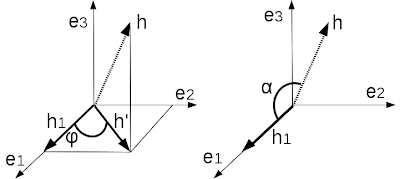## Abstract

When I compute something, I see it is correct, but sometimes my intuition doesn't work. Especially, I am not good at statistics, though, sometimes I don't see geometry also. I can show you such example this time.

## Vector projection and directional cosine

My friend Dietger asked me a problem. Figure 1 shows the problem. Let $$\mathbf{h}'$$ is a projected vector of $$\mathbf{h}$$ on the $$\mathbf{e}_1,\mathbf{e}_2$$ plane of an orthogonal coordinate system. Where $$\mathbf{h}$$ is an arbitrary unit vector. Let $$\mathbf{h}_1$$ is the projection of $$\mathbf{h}$$ on the axis $$\mathbf{e}_1$$. Then show
$\cos \alpha = |\mathbf{h}'| \cos \phi.$
It's intuitively odd for me that there is a length ratio $$h'$$ between $$\cos \alpha$$ and $$\cos \phi$$.Figure 1. Two projections of an unit vector $$\mathbf{h}$$.

However, these are projections, therefore some $$\cos$$  relationships. Let's start with  $$\mathbf{h}_1$$.  $$\mathbf{h}_1$$ is a projection of  $$\mathbf{h}$$ on $$\mathbf{e}_1$$ axis,
\begin{eqnarray*}
|\mathbf{h}_1| &=&  \mathbf{h}\cdot\mathbf{e}_1\\
&=& |\mathbf{h}| |\mathbf{e}_1| \cos \alpha \\
&=& \cos \alpha.
\end{eqnarray*}
If you noticed $$\mathbf{h}_1$$ is a projection of $$\mathbf{h}'$$ on $$\mathbf{e}_1$$ axis,
\begin{eqnarray*}
\mathbf{h}'\cdot\mathbf{e}_1 &=& |\mathbf{h}'||\mathbf{e}_1| \cos \phi \\
&=& |\mathbf{h}'| \cos \phi.
\end{eqnarray*}
This is actually $$|\mathbf{h}_1|$$. See the Figure 2. Therefore,
\begin{eqnarray*}
\cos \alpha &=& |\mathbf{h}'| \cos \phi.
\end{eqnarray*}Figure 2. The relationship among $$\mathbf{h}$$, $$\mathbf{h}'$$ and $$\mathbf{h}_1$$.
In the end, if you noticed $$\mathbf{h}_1$$ is a direct projection of $$\mathbf{h}$$ or projection of projection, there is no mystery here.

When I understand this problem as above, I recall directional cosine.  I learned this long time ago, but this idea is located nowhere in my head and no connection with other mathematical ideas. I just remember the squared sum of the cosines becomes 1. It's a kind of left out idea for me. But, if the projection is on the each axis, this is directional cosine. Then the squared sum is of course one. (Or the square root of squared sum is one. But square root of one is anyway one.) Because this is the length of unit vector, which is one. It's so simple. I don't know why I never realize the directional cosine is just coordinate elements of unit vector.

### MathJax Test

It's bit cumbersome to create an image for each equation every time. So I try MathJax here. This is a great software!

<script type="text/javascript"

$|\mathbf{h}_1| = \mathbf{h}\cdot \mathbf{e}_1 = |\mathbf{h}| |\mathbf{e}_1| \cos \alpha = \cos \alpha$
becomes $|\mathbf{h}_1| = \mathbf{h}\cdot\mathbf{e}_1 = |\mathbf{h}| |\mathbf{e}_1| \cos \alpha = \cos \alpha$ Awesome.